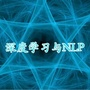Lots of learning tasks require dealing with graph data which contains rich relation information among elements. Modeling physics system, learning molecular fingerprints, predicting protein interface, and classifying diseases require that a model to learn from graph inputs. In other domains such as learning from non-structural data like texts and images, reasoning on extracted structures, like the dependency tree of sentences and the scene graph of images, is an important research topic which also needs graph reasoning models. Graph neural networks (GNNs) are connectionist models that capture the dependence of graphs via message passing between the nodes of graphs. Unlike standard neural networks, graph neural networks retain a state that can represent information from its neighborhood with an arbitrary depth. Although the primitive graph neural networks have been found difficult to train for a fixed point, recent advances in network architectures, optimization techniques, and parallel computation have enabled successful learning with them. In recent years, systems based on graph convolutional network (GCN) and gated graph neural network (GGNN) have demonstrated ground-breaking performance on many tasks mentioned above. In this survey, we provide a detailed review over existing graph neural network models, systematically categorize the applications, and propose four open problems for future research.0+33+下载关闭预览

相关内容0+2+0+0+8+0+0+14+0+1+16+0+2+35+0+Both generative adversarial network models and variational autoencoders have been widely used to approximate probability distributions of datasets. Although they both use parametrized distributions to approximate the underlying data distribution, whose exact inference is intractable, their behaviors are very different. In this report, we summarize our experiment results that compare these two categories of models in terms of fidelity and mode collapse. We provide a hypothesis to explain their different behaviors and propose a new model based on this hypothesis. We further tested our proposed model on MNIST dataset and CelebA dataset.0+5+0+下载预览2+11+0+0+19+0+0+18+0+
Top